## Excel WEIBULL.DIST Function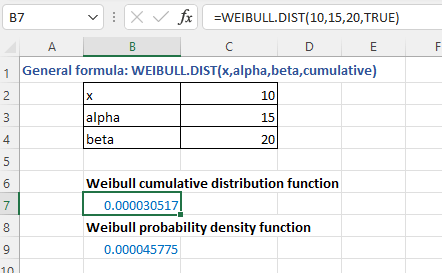### Description

The Excel WEIBULL.DIST function returns the Weibull probability density function or Weibull cumulative distribution for a supplied set of parameters. It is frequently used for reliability analysis, e.g., for testing the mean time of the failure of an equipment in a specific time range.

Formula syntax

WEIBULL.DIST(x_range,alpha,betta,cumulative)

Arguments

 x: Required, the value at which to evaluate the function. alpha: Required, a parameter of the distribution. beta: Required, a parameter of the distribution. cumulative: Required, a logical value that determines which form of the function to be used. TRUE: Weibull cumulative distribution function is used;< FALSE: Weibull probability density function is used.

### Returns

WEIBULL.DIST function returns a numeric value.

### Remarks

1. The function returns #VALUE! error value if x, alpha, or beta is a non-numeric value.

2. The function returns #NUM! error value when

• x is negative;
• alpha or beta ≤ 0
• X_range and prob_range have different numbers of data points, WEIBULL.DIST function return the #N/A error value.

3. The equation for Weibull cumulative distribution function is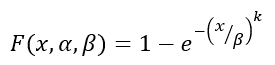4. The equation for Weibull probability density function is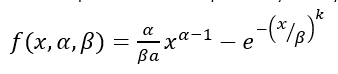5. When alpha = 1, WEIBULL.DIST function returns the exponential distribution with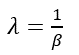### Version

Excel 2010 and later versions

### Usage and Examples

Basic usage

For getting the Weibull cumulative distribution function (cumulative as TURE) and Weibull probability density function (cumulative as FALSE) separately for the supplied x (10), alpha (15),beta (20), you can use the WEIBULL.DIST function as these:

=WEIBULL.DIST(10,15,20,TRUE) // Weibull cumulative distribution function

=WEIBULL.DIST(10,15,20,FALSE) // Weibull probability density function

Press Enter key to get the result.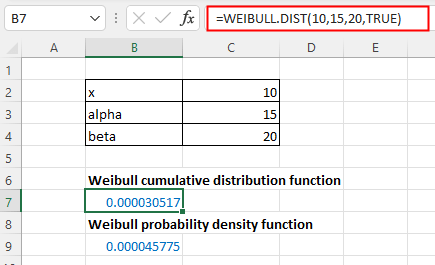#### Other Functions:

• Excel POISSON Function
The Excel POISSON function returns the Poisson distribution which is used to predict the number of events occurring over a specific time.

• Excel WEIBULL Function
The Excel WEIBULL function returns the Weibull probability density function or Weibull cumulative distribution for a supplied set of parameters.

• Excel EXPON.DIST Function
EXPON.DIST function used in Excel 2010, returns the exponential distribution based on the given x value and the parameter of the distribution.

• Excel F.DIST Function
The Excel F.DIST function returns the F probability distribution which is usually used to measure the degree of diversity between two data sets.

### The Best Office Productivity Tools

#### Kutools for Excel - Helps You To Stand Out From Crowd

 Popular Features: Find, Highlight or Identify Duplicates  |  Delete Blank Rows  |  Combine Columns or Cells without Losing Data  |  Round without Formula ... Super VLookup: Multiple Criteria  |  Multiple Value  |  Across Multi-Sheets  |  Fuzzy Lookup... Adv. Drop-down List: Easy Drop Down List  |  Dependent Drop Down List  |  Multi-select Drop Down List... Column Manager: Add a Specific Number of Columns  |  Move Columns  |  Toggle Visibility Status of Hidden Columns  |  Compare Columns to Select Same & Different Cells ... Featured Features: Grid Focus  |  Design View  |  Big Formula Bar  |  Workbook & Sheet Manager | Resource Library (Auto Text)  |  Date Picker  |  Combine Worksheets  |  Encrypt/Decrypt Cells  |  Send Emails by List  |  Super Filter  |  Special Filter (filter bold/italic/strikethrough...) ... Top 15 Toolsets:  12 Text Tools (Add Text, Remove Characters ...)  |  50+ Chart Types (Gantt Chart ...)  |  40+ Practical Formulas (Calculate age based on birthday ...)  |  19 Insertion Tools (Insert QR Code, Insert Picture from Path ...)  |  12 Conversion Tools (Numbers to Words, Currency Conversion ...)  |  7 Merge & Split Tools (Advanced Combine Rows, Split Excel Cells ...)  |  ... and more

Kutools for Excel Boasts Over 300 Features, Ensuring That What You Need is Just A Click Away...#### Office Tab - Enable Tabbed Reading and Editing in Microsoft Office (include Excel)

• One second to switch between dozens of open documents!
• Reduce hundreds of mouse clicks for you every day, say goodbye to mouse hand.
• Increases your productivity by 50% when viewing and editing multiple documents.
• Brings Efficient Tabs to Office (include Excel), Just Like Chrome, Edge and Firefox.